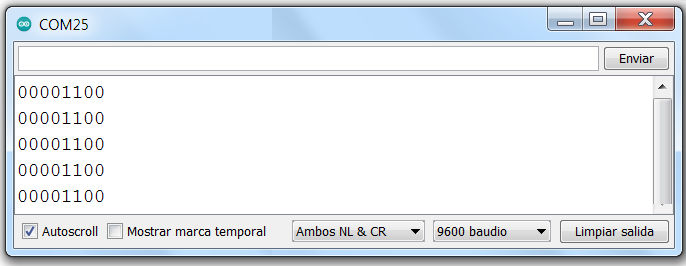# I want to send binary to Arduino

Hello this is my first app and I am trying to make a simple program. I want to control 48 individual relays hooked up with an arduino with by 48 buttons on mit app inventor. Every method I have used to send binary has resulted in the arduino receiving a totally differant binary number.

I have tried to just send text but once I get to double digits it see 12 as 1 and 2

Convert each byte to a decimal number, put them all in a list, and send the list as unsigned bytes.

Send the number as text and have the Arduino Sketch convert it to binary.

borrart_BT_Binario.aia (9.4 KB)

``````void setup() {
Serial.begin(9600); // Inicializar la comunicación serial a 9600 baudios
}

void loop() {
if (Serial.available()) {

// Convertir el número a binario
String binary = String(num, BIN);
while (binary.length() < 8) {
binary = "0" + binary; // Fill 0 left
}

Serial.println(binary); // Print binary number.
}
}
``````If what you want is to obtain position 12 in the output:

``````void setup() {
Serial.begin(9600); // Inicializar la comunicación serial a 9600 baudios
}

void loop() {
if (Serial.available()) {
int num = Serial.parseInt(); // Leer el número recibido como texto

// Convertir el número a binario
String binary = "";
for (int i = 0; i < 48; i++) {
if (i == num) {
binary += "1"; // Agregar "1" en la posición correspondiente al número
} else {
binary += "0"; // Agregar "0" en las demás posiciones
}
}

Serial.println(binary); // Imprimir el número binario
}
}
``````

``````if (Serial1.available()) {
int blue = Serial1.parseInt();
if (blue == 1) {
led1.high();
eprom1 =1;
myMem.put(1, eprom1);
Serial1.println("drita1 uardes ON");
}

if (blue == 2) {
eprom1=0;
led1.low();
myMem.put(1, eprom1);
Serial1.println("drita1 uardes OFF");
}
``````

pcs of my code thanks to Juan Antonio
[Bluetooth HC-06. Arduino. Send. Receive. Send text file. Multitouch. Image - #19 by Juan_Antonio](pcs of my code thanks to Juan Antonio Bluetooth HC-06. Arduino. Send. Receive. Send text file. Multitouch. Image - #19 by Juan_Antonio)